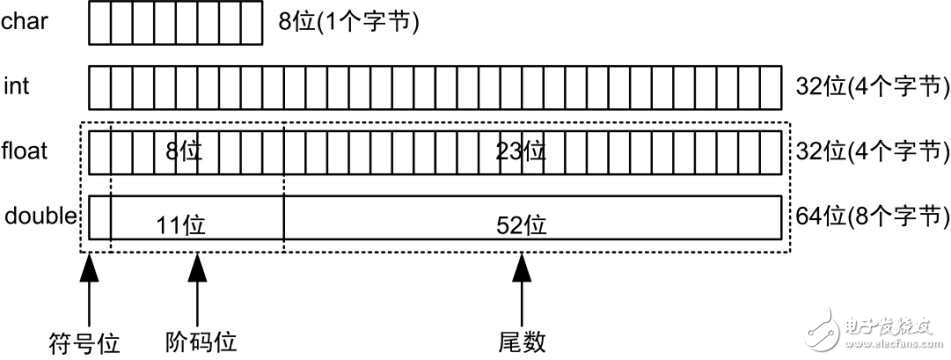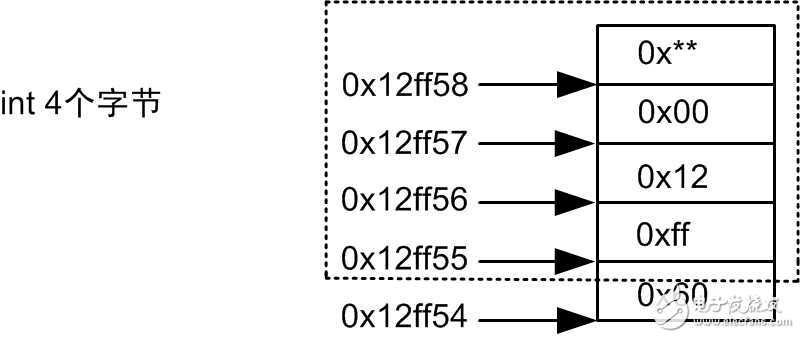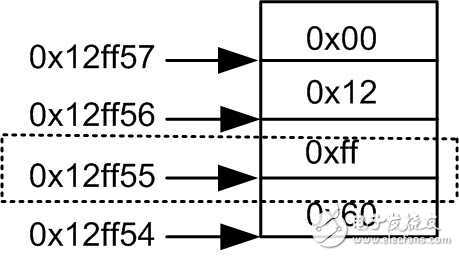# C语言面试（二）总结

#include<stdio.h>
static int a;
static int b[];
int main(int argc,char *argv[])
{
printf("%d %d\n",a,b);
return 0;
}
static int a = 8;
static int b;

static int a ,这句程序是声明全局变量a;static int b[],这句程序是声明全局数组变量b,并且是不完全声明，也

#include<stdio.h>
static int a = 8 ;
int main( int argc , char *argv[] )
{
int a = 4 ;
printf( "%d \n" , a ) ;
return 0 ;
} 

C语言规定，局部变量在自己的可见范围内会“挡住”同名的全局变量，让同名的全局变量临时不可见。即在局部变量的可见范围内不能访问同名的全局变量。因此本程序输出为：4。
char、int、float、double的数据存储

float i = 3 ;
int   j = *(int*)(&i) ;
printf( "i = %f \n" , i ) ;
printf( "j = %#x \n" , j ) ;


i是毋庸置疑是：3.000000。但是j呢？3.000000？答案是否定的，j是输出：0x4040 0000。有人会问了，难道j是随机输出？瞎说，j输出0x4040 0000是有依据，是一个定值！

char、int、float、double的存储方式如下：

i = -3.500000
j = 0xc0600000

unsigned int b = 0x12ff60 ;
printf("( (int)(&b)+1 )         = %#x \n" , ( (int)(&b)+1 )           ) ;
printf("*( (int*)( (int)(&b)+1 ) )  = %#x \n" , *( (int*)( (int)(&b)+1 ) )   ) ;
printf("*( (char*)( (int)(&b)+1 ) ) = %#x \n" , *( (char*)( (int)(&b)+1 ) )  ) ;

printf("*( (char*)( (int)(&b)+1 ) ) = %d \n" , *( (char*)( (int)(&b)+1 ) ) ) ;

printf("*( (char*)( (int)(&b)+1 ) ) = %#x \n" , *( (char*)( (int)(&b)+1 ) ) ) ;
 f("*( (unsigned char*)( (int)(&b)+1 ) ) = %#x \n" ,
*( (unsigned char*)( (int)(&b)+1 ) ) ) ;